# Applying Research

### Introduction

This page explains how to you can use the Research Environment to develop and test a Airline Buybacks hypothesis, then put the hypothesis in production.

### Create Hypothesis

Buyback represents a company buy back its own stocks in the market, as (1) management is confident on its own future, and (2) wants more control over its development. Since usually buyback is in large scale on a schedule, the price of repurchasing often causes price fluctuation.

Airlines is one of the largest buyback sectors. Major US Airlines use over 90% of their free cashflow to buy back their own stocks in the recent years. Therefore, we can use airline companies to test the hypothesis of buybacks would cause price action. In this particular exmaple, we're hypothesizing that difference in buyback price and close price would suggest price change in certain direction. (we don't know forward return would be in momentum or mean-reversion in this case!)

### Import Libraries

We'll need to import libraries to help with data processing, validation and visualization. Import SmartInsiderTransaction class, statsmodels, sklearn, numpy, pandas and seaborn libraries by the following:

from QuantConnect.DataSource import SmartInsiderTransaction

from statsmodels.discrete.discrete_model import Logit
from sklearn.metrics import confusion_matrix
import numpy as np
import pandas as pd
import seaborn as sns

### Get Historical Data

To begin, we retrieve historical data for researching.

1. Instantiate a QuantBook.
2. qb = QuantBook()
3. Select the airline tickers for research.
4. assets = ["LUV",   # Southwest Airlines
"DAL",   # Delta Airlines
"UAL",   # United Airlines Holdings
"AAL",   # American Airlines Group
"SKYW",  # SkyWest Inc.
"ALGT",  # Allegiant Travel Co.
"ALK"    # Alaska Air Group Inc.
]
5. Call the AddEquity method with the tickers, and its corresponding resolution. Then call AddData with SmartInsiderTransaction to subscribe to their buyback transaction data. Save the Symbols into a dictionary.
6. symbols = {}
for ticker in assets:
symbols[symbol] = qb.AddData(SmartInsiderTransaction, symbol).Symbol

If you do not pass a resolution argument, Resolution.Minute is used by default.

7. Call the History method with a list of Symbols for all tickers, time argument(s), and resolution to request historical data for the symbols.
8. history = qb.History(list(symbols.keys()), datetime(2019, 1, 1), datetime(2021, 12, 31), Resolution.Daily)
9. Call SPY history as reference.
10. spy = qb.History(qb.AddEquity("SPY").Symbol, datetime(2019, 1, 1), datetime(2021, 12, 31), Resolution.Daily)
11. Call the History method with a list of SmartInsiderTransaction Symbols for all tickers, time argument(s), and resolution to request historical data for the symbols.
12. history_buybacks = qb.History(list(symbols.values()), datetime(2019, 1, 1), datetime(2021, 12, 31), Resolution.Daily)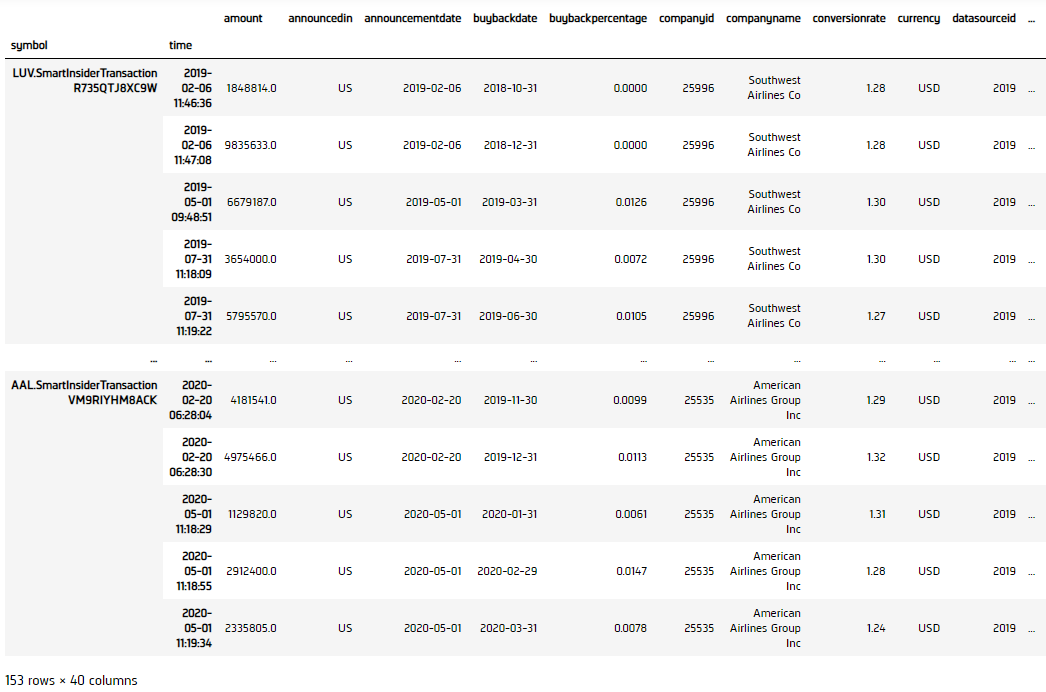### Prepare Data

We'll have to process our data to get the buyback premium/discount% vs forward return data.

1. Select the close column and then call the unstack method.
2. df = history['close'].unstack(level=0)
spy_close = spy['close'].unstack(level=0)
3. Call pct_change to get the daily return of close price, then shift 1-step backward as prediction.
4. ret = df.pct_change().shift(-1).iloc[:-1]
ret_spy = spy_close.pct_change().shift(-1).iloc[:-1]
5. Get the active forward return.
6. active_ret = ret.sub(ret_spy.values, axis=0)
7. Select the ExecutionPrice column and then call the unstack method to get the buyback dataframe.
8. df_buybacks = history_buybacks['executionprice'].unstack(level=0)
9. Convert buyback history into daily mean data.
10. df_buybacks = df_buybacks.groupby(df_buybacks.index.date).mean()
df_buybacks.columns = df.columns
12. df_close = df.reindex(df_buybacks.index)[~df_buybacks.isna()]
df_buybacks = (df_buybacks - df_close)/df_close
13. Create a Dataframe to hold the buyback and 1-day forward return data.
14. data = pd.DataFrame(columns=["Buybacks", "Return"])
15. Append the data into the Dataframe.
16. for row, row_buyback in zip(active_ret.reindex(df_buybacks.index).itertuples(), df_buybacks.itertuples()):
index = row
data = pd.concat([data, pd.DataFrame({"Buybacks": row_buyback[i], "Return":row[i]}, index=[index])])
17. Call dropna to drop NaNs.
18. data.dropna(inplace=True)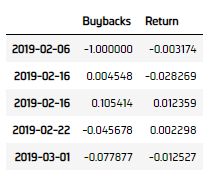### Test Hypothesis

We would test (1) if buyback has statistically significant effect on return direction, and (2) buyback could be a return predictor.

1. Get binary return (+/-).
2. binary_ret = data["Return"].copy()
binary_ret[binary_ret < 0] = 0
binary_ret[binary_ret > 0] = 1
3. Construct a logistic regression model.
4. model = Logit(binary_ret.values, data["Buybacks"].values).fit()
5. Display logistic regression results.
6. display(model.summary())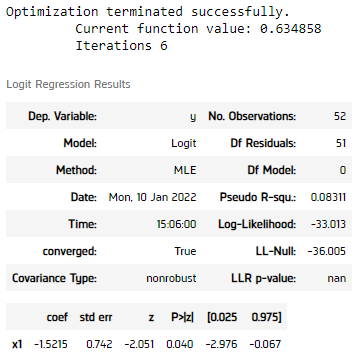We can see a p-value of < 0.05 in the logistic regression model, meaning the separation of positive and negative using buyback premium/discount% is statistically significant.

7. Plot the results.
8. plt.figure(figsize=(10, 6))
sns.regplot(x=data["Buybacks"]*100, y=binary_ret, logistic=True, ci=None, line_kws={'label': " Logistic Regression Line"})
plt.plot([-50, 50], [0.5, 0.5], "r--", label="Selection Cutoff Line")
plt.xlim([-50, 50])
plt.ylabel("Profit/Loss")
plt.legend()
plt.show()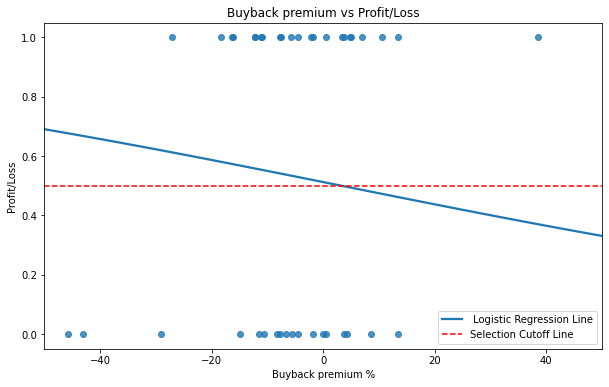Interesting, from the logistic regression line, we observe that when the airlines brought their stock in premium price, the price tended to go down, while the opposite for buying back in discount.

Let's also study how good is the logistic regression.

9. Get in-sample prediction result.
10. predictions = model.predict(data["Buybacks"].values)
for i in range(len(predictions)):
predictions[i] = 1 if predictions[i] > 0.5 else 0
11. Call confusion_matrix to contrast the results.
12. cm = confusion_matrix(binary_ret, predictions)
13. Display the result.
14. df_result = pd.DataFrame(cm,
index=pd.MultiIndex.from_tuples([("Prediction", "Positive"), ("Prediction", "Negative")]),
columns=pd.MultiIndex.from_tuples([("Actual", "Positive"), ("Actual", "Negative")]))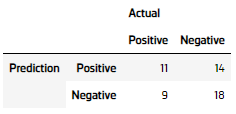The logistic regression is having a 55.8% accuracy (55% sensitivity and 56.3% specificity), this can suggest a > 50% win rate before friction costs, proven our hypothesis.

### Set Up Algorithm

Once we are confident in our hypothesis, we can export this code into backtesting. One way to accomodate this model into backtest is to create a scheduled event which uses our model to predict the expected return.

def Initialize(self) -> None:

#1. Required: Five years of backtest history
self.SetStartDate(2017, 1, 1)

#2. Required: Alpha Streams Models:
self.SetBrokerageModel(BrokerageName.AlphaStreams)

#3. Required: Significant AUM Capacity
self.SetCash(1000000)

#4. Required: Benchmark to SPY
self.SetBenchmark("SPY")

self.SetPortfolioConstruction(EqualWeightingPortfolioConstructionModel())
self.SetExecution(ImmediateExecutionModel())

# Set our strategy to be take 5% profit and 5% stop loss.

# Select the airline tickers for research.
self.symbols = {}
assets = ["LUV",   # Southwest Airlines
"DAL",   # Delta Airlines
"UAL",   # United Airlines Holdings
"AAL",   # American Airlines Group
"SKYW",  # SkyWest Inc.
"ALGT",  # Allegiant Travel Co.
"ALK"    # Alaska Air Group Inc.
]

# Call the AddEquity method with the tickers, and its corresponding resolution. Then call AddData with SmartInsiderTransaction to subscribe to their buyback transaction data.
for ticker in assets:

# Initialize the model
self.BuildModel()

# Set Scheduled Event Method For Our Model Recalibration every month
self.Schedule.On(self.DateRules.MonthStart(), self.TimeRules.At(0, 0), self.BuildModel)

# Set Scheduled Event Method For Trading
self.Schedule.On(self.DateRules.EveryDay(), self.TimeRules.BeforeMarketClose("SPY", 5), self.EveryDayBeforeMarketClose)

We'll also need to create a function to train and update the logistic regression model from time to time.

def BuildModel(self) -> None:
qb = self
# Call the History method with list of tickers, time argument(s), and resolution to request historical data for the symbol.
history = qb.History(list(self.symbols.keys()), datetime(2015, 1, 1), datetime.now(), Resolution.Daily)

# Call SPY history as reference
spy = qb.History(["SPY"], datetime(2015, 1, 1), datetime.now(), Resolution.Daily)

# Call the History method with list of buyback tickers, time argument(s), and resolution to request buyback data for the symbol.
history_buybacks = qb.History(list(self.symbols.values()), datetime(2015, 1, 1), datetime.now(), Resolution.Daily)

# Select the close column and then call the unstack method to get the close price dataframe.
df = history['close'].unstack(level=0)
spy_close = spy['close'].unstack(level=0)

# Call pct_change to get the daily return of close price, then shift 1-step backward as prediction.
ret = df.pct_change().shift(-1).iloc[:-1]
ret_spy = spy_close.pct_change().shift(-1).iloc[:-1]

# Get the active return
active_ret = ret.sub(ret_spy.values, axis=0)

# Select the ExecutionPrice column and then call the unstack method to get the dataframe.

# Convert buyback history into daily mean data

# Create a dataframe to hold the buyback and 1-day forward return data

# Append the data into the dataframe
index = row

# Call dropna to drop NaNs
data.dropna(inplace=True)

# Get binary return (+/-)
binary_ret = data["Return"].copy()
binary_ret[binary_ret < 0] = 0
binary_ret[binary_ret > 0] = 1

# Construct a logistic regression model
self.model = Logit(binary_ret.values, data["Buybacks"].values).fit()

Now we export our model into the scheduled event method. We will switch qb with self and replace methods with their QCAlgorithm counterparts as needed. In this example, this is not an issue because all the methods we used in research also exist in QCAlgorithm.

def EveryDayBeforeMarketClose(self) -> None:
qb = self
# Get any buyback event today

# Select the ExecutionPrice column and then call the unstack method to get the dataframe.

# Convert buyback history into daily mean data

# ==============================

insights = []

# Iterate the buyback data, thne pass to the model for prediction
for i in range(len(row)):
prediction = self.model.predict(row[i])

# Long if the prediction predict price goes up, short otherwise. Do opposite for SPY (active return)
if prediction > 0.5:
insights.append( Insight.Price(row.index[i].split("."), timedelta(days=1), InsightDirection.Up) )
insights.append( Insight.Price("SPY", timedelta(days=1), InsightDirection.Down) )
else:
insights.append( Insight.Price(row.index[i].split("."), timedelta(days=1), InsightDirection.Down) )
insights.append( Insight.Price("SPY", timedelta(days=1), InsightDirection.Up) )

self.EmitInsights(insights)

### Reference

• US Airlines Spent 96% of Free Cash Flow on Buybacks: Chart. B. Kochkodin (17 March 2020). Bloomberg. Retrieve from: https://www.bloomberg.com/news/articles/2020-03-16/u-s-airlines-spent-96-of-free-cash-flow-on-buybacks-chart.

### Clone Example Project

You can also see our Videos. You can also get in touch with us via Discord.